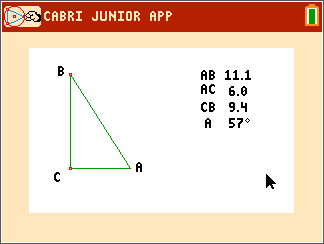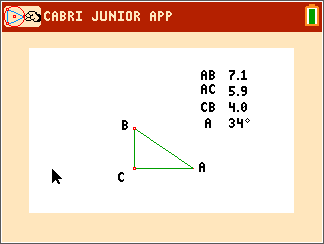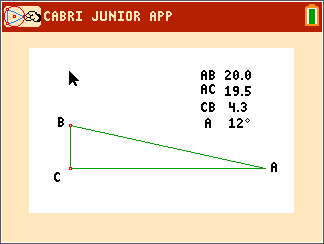# Activities

••• ##### Subject Area

• Math: Precalculus: Trigonometry (Triangle and Circular Functions)

• ##### Author9-12

45 Minutes

• ##### Device
• TI-84 Plus
• TI-84 Plus Silver Edition
•TI-84 Plus C Silver Edition
•TI-84 Plus CE
• ##### Software

TI Connect™ CE

## Trig Ratios#### Activity Overview

Students will use Cabri™ Jr. to discover the relationship between the trigonometric functions: sine, cosine and tangent and the side length ratios of a right triangle.

#### Key Steps

•Students use Cabri™ Jr. to find the relationships between ratios of the side lengths and the trigonometric functions.
They find the sine, cosine, and tangent of an angle then find the ratio between the hypotenuse, adjacent and opposite sides.

•Students then form a hypothesis about the relationships they observe between the trigonometric values and the side lengths.